## PROPERTIES - Index

1.    Density
DENSITY:
A hydrometer is an instrument to measure the density of a liquid in relation to water (specific gravity).The bulb pulls the liquid into the hydrometer (see figure below) and the notches on the glass float are values of the specific gravity.For example, if water were measured the specific gravity would be 1.000.
##### TEMPERATURE CORRECTION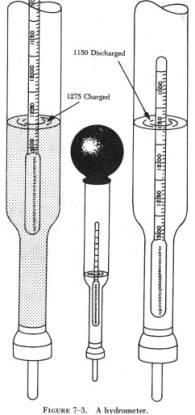Most hydrometers are calibrated at 60°F and sample temperatures deviating from this need correction.The chart below contains the correction factors to add to the specific gravity reading.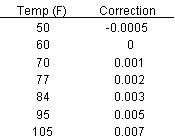The density can also be simply measured using a volumetric flask and mass balance.

DENSITY OF MAGNETIC FLUIDS

Experimental results, like those in the comparison table, show that the ferrofluid has a higher density than its carrier fluid.Density increases as the volume fraction of solid particles increases in the colloidal mixture.If the volume of the colloidal mixture is assumed to be the same as the sum of all the volumes of the components, the density is represented as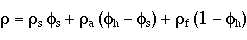In our case, the density of the solid phase is greater than either the surfactant or carrier fluid.Thus, an increase in the volume fraction of the solid phase will cause an increase in the overall density.

If we further assume that the density of the surfactant and carrier fluid are approximately the same the previous equation reduces to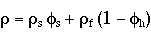A common way to find the volume fraction of the solid phase is using this equation.

 NOTATION Greek Letters  r=density  f=volume fraction  fn=hydrodynamic volume concentration Subscripts  s=solid (magnetic) phase  a=surfactant  f=carrier liquid

HEAT CAPACITY:

Heat capacity is determined by a calorimeter.It can be deduced from the equation E=mCpDT.E is the energy put into the calorimeter, m is the mass of sample in the calorimeter, Cp is the heat capacity, and DT is the change in temperature.Data is obtained such that Cp is the only unknown in this equation.

The mass (m) of the test liquid put into the calorimeter is known.The energy (E) is determined by multiplying the potential by current over the time it runs (E=V*I*t).Temperature rise (DT) is recorded via the thermometer.

HEAT CAPACITY OF FERROFLUIDS

Assuming the molecular interactions greatly exceed those of particle-particle the heat capacity can be represented using the mixing rule.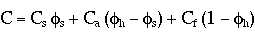In our case the heat capacity of the magnetic solid is much less than that of the fluid or surfactant.Thus, an increase in mass concentration of the solid particles will cause the overall heat capacity of the ferrofluid to decline.

Berkovsky mentions that the validity of this expression is backed by experimental data.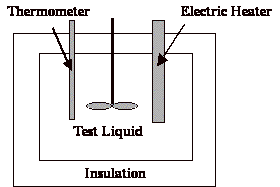NOTATION C=heat capacity (specific heat)  Y=mass concentration Subscripts  m=magnetite  a=stabilizer  f=liquid base

THERMAL CONDUCTIVITY:

Hot wire technique uses two wires to measure the conductivity.One generates thermal energy and the other is used as a thermocouple.They are placed a known distance apart in a sample and are not moved.Once the system is started the temperature is recorded vs time at a constant q output from the heater.The conductivity is determined from the plot DT vs. t.

THERMAL CONDUCTIVITY OF FERROFLUIDS

When we add magnetic particles to a carrier fluid the conductivity of the mixture increases.This is expected because the thermal conductivity of the magnetic particles being added can have 10-100 times higher conductivities than the carrier fluid.Obviously, since the conductivity of the magnetite is so high compared to that of the carrier liquid, increases in its volume fraction are bound to have a dramatic effect.

Experimental data of conductivity vs. volume fraction of the solid phase yields a straight line.(See Berkovsky (1993) pg. 49.)This line, empirically fit to the data, yields a maximum error of 5%.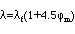Berkovsky suggests that when lf<<lm and fs<<1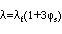be used.No information was found regarding how smallfs or lf compared to lm should be.

 NOTATION l=thermal conductivity Subscripts  s=solid (magnetic) phase  a=surfactant  f=carrier liquid

VISCOSITY

The viscosity of a magnetic fluid depends on the solid fraction f, the viscosity of the carrier fluid h0 and the applied magnetic field (orientation as well as force).

For the determination without an applied magnetic field every standard viscometer can be used. In addition the viscosity can be estimated using one of the following formulas (from Rosensweig, ãFerrohydrodynamicsä):

small particle concentration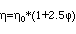higher particle concentration: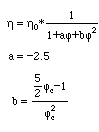fc = 0.74 (closed package)

Experiments to determine the viscosity with an applied magnetic field where conducted by McTague (1969) with a capillary tube viscometer. The result of this experiments is shown in the figure below and can be expressed with the following formulas: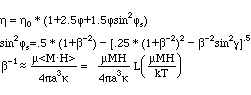L: Langevin function

g : angle between axis of rotation of the fluid and H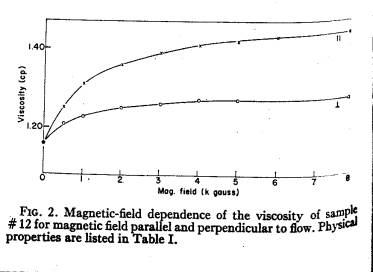McTague, J. Chem. Phys., vol. 51, no. 1, 1969, p. 133-6

VORTEX / ROTATIONAL VISCOSITY

The rotational or spin viscosity of a ferrofluid describes the dampening through internal rotational friction. It can be measured using a torsional pendulum by determination of the
resulting deflection amplitude for different frequencies.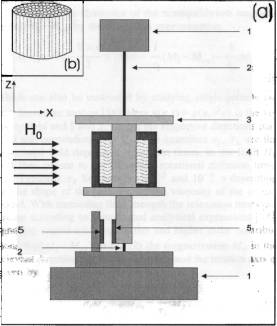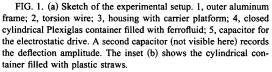Due to Embs, Muller, Wagner, Knorr, and Lucke, Phys. Rev. E, 61, p.R2196 (2000).

Furthermore it can be estimated using the following equation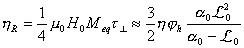`or be estimated with the following equation derived by Shliomis (1972):`
`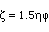`

THERMAL EXPANSION COEFFIZIENT

Definition: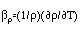Typical value

Shell oil7.17 ´10-4

Ferrofluid7.17´10-4

Measurement method

Use the instruments such as following to measure it directly.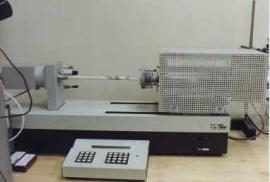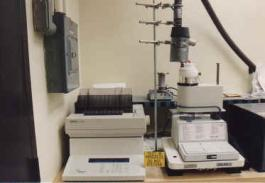Linear Thermal Expansion measurements from -80°C to +1600°C in air, argon, nitrogen or vacuum.

Calculation from the properties of its components: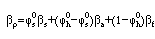where bs, ba, bf are the thermal expansion coefficients of particles, a stabilizer and a carrier-liquid.jis volume fraction.

PYROMAGNETIC COEFFICIENT

## Definition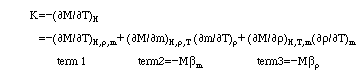WhereMmagnetization of magnetic fluids;

Hmagnetic strength;

Tabsolute temperature;

mmagnetic moment of a particle;

Typical value

In the case of strong fields(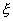>>1), the fluid is in a state of magnetic saturation and despite the Brownian movement all the magnetic moments of particles are aligned along a magnetic field. Therefore, the Brownian movement doesnât not contribute to the temperature dependence of magnetization and the first term in above equation is equal to zero. Then, for a pyromagnetic coefficient: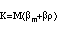Far from the Curie temperature the quantity bm is very small and for magnetic fluids it may be neglected. This implies the pyromagnetic coefficient of the magnetic fluid, being in the saturation state, is determined only by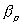For most situations,

~(1-100) A (mK)-1

In the case (<<1),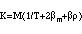At the room temperature, it has the order of 3´10-3K-1

FerrofluidK=12;

Measurement method

TIME CONSTANT

Definition

The time of onset of equilibrium concentration is determined by the characteristic time of diffusion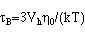MAGNETIC SUSCEPTIBILITY

Magnetic susceptibility is a quantitative measure of the extent to which a material may be magnetized in relation to a given applied magnetic field. The magnetic susceptibility of a material, commonly symbolized by c, is equal to the ratio of themagnetization M within the material to the applied magnetic field strength H, or c = M/H. This ratio, strictly speaking, is the volume susceptibility, because magnetization essentially involves a certain measure of magnetism (dipole moment) per unit volume.

Magnetic materials may be classified as diamagnetic, paramagnetic, or ferromagnetic on the basis of their susceptibilities.

Ferromagnetic materials, such as iron and cobalt, do not have constant susceptibilities; the magnetization is not usually proportional to the applied field strength. Measured ferromagnetic susceptibilities have relatively large positive values, sometimes in excess of 1,000. Thus, within ferromagnetic materials, the magnetization may be more than 1,000 times larger than the external magnetizing field, because such materials are composed of highly magnetized clusters of atomic magnets (ferromagnetic domains) that are more easily lined up by the external field.